Mandelbrot setThe Mandelbrot set is one of the most celebrated objects in nonlinear dynamics. Let us consider a sequence of complex numbers zn defined by a recurrent relation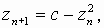where c is a complex parameter, with an initial condition z0=0. At small values of ± the sequence will be bounded, for larger ones it will diverge.

The set of ± on the complex plane, at which the sequence zn is bounded, is called the Mandelbrot set.

It is named after B.Mandelbrot, the author of the book ōFractal Geometry of Natureö. After publication In 1985 in a section of amateur science in ōScientific Americanö it becomes very known and popular. For example, it was used as an emblem of the Soros Foundation.

Mandelbrot set as an example of a fractal

Fractals are sets manifesting self-similarity in exact or approimate sense on different levels of resolution of their geometric structure. This term is used also for objects in nature, which possess this property in a wide range of scales.

The Mandelbrot set is a commonly known example of fractals. Below you can see a set of pictures of this set with a resolution growing step by step.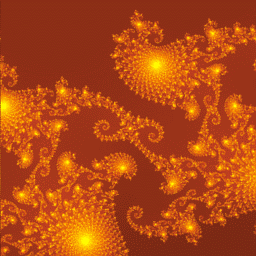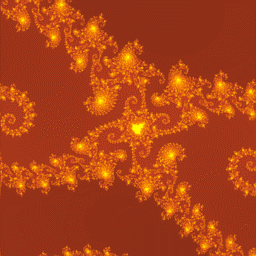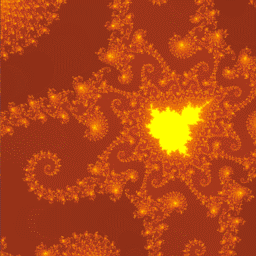Dynamics of complex analytic maps

The Mandelbrot fractal is only one representative of the remarkable family of objects studied in a buetiful subfield of nonlinear science, the dynamics of iterative complex analytic maps. On the plane of complex variable z at each given parameter value c there is a set of points, starting from which the orbit never diverges; the border of this is called the Julia set (see diagrams on periphery of the figure below).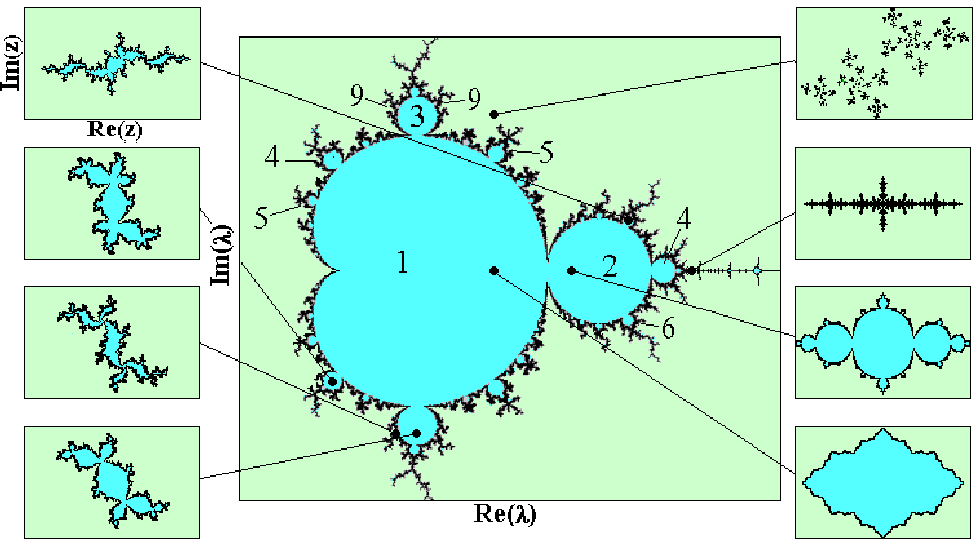An interesting question:
Do these objects generated by abstract mathematical procedures have any relation to dynamics of real physical systems?

Dynamics of physical systems are described most often in terms of differential equations. Its reformulation in terms of maps may be performed with a use of the construction called the Poincare cross-section. If the phase space is three-dimensional, this procedure produces a two-dimensional Poincare map. This is illustrated below by two figures, the first one relates to an autonomous system of the 3-d order, and the second one to a periodically driven system of the 2-nd order (to say, an oscillator under periodic force).If we take a complex analytic map zn+1=f(zn) and separate the real and imaginary parts, the resulting will be a two-dimensional map in real domain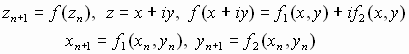It belongs to a very special class of maps, the maps satisfying the Cauchy - Riemann equations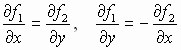Is it possible to invent a physical system to have the Poincare map belonging the Cauchy - Riemann class of maps? (And, moreover, to ensure it to be close to the quadratic map producing the Mandelbrot set.) For sure, it is impossible for a system with 3D phase space. What about higher dimensions? Apparently, the problem is nontrivial: The required properties of the map are so special!

[One attempt to construct a system of the desirable type is discussed in a reference:
C.Beck. Physical meaning for Mandelbrot and Julia sets. Physica D125, 1999, 171.]

It is known that presence of the Mandelbrot set in complex plane is associated with presence of the period-doubling bifurcation cascade on the real axis (see the figure below).Period doubling cascade is a universal scenario of transition to chaos, which occurs in many nonlinear dissipative systems. An example is a driven dissipative oscillator with quadratic nonlinearity:Figure below shows a set of phase portraits obtained at g=0.2, F=0.23 for several values of l.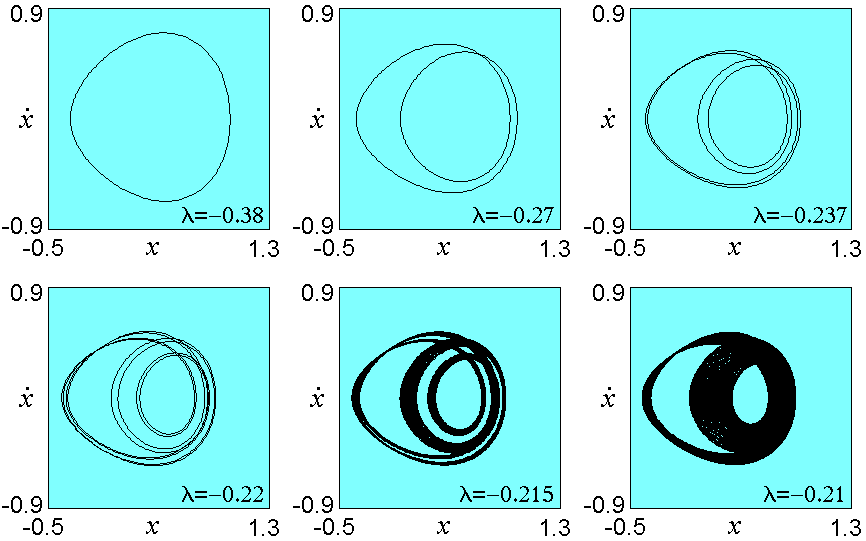Our idea is to use such objects as building blocks in a construction of systems demonstrating the phenomena of complex analytic dynamics. Let us explain it, first, on the simplest example of complex quadratic map.

From complex map to coupled systems

In the complex equation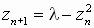let us set z=x+iy, l=l'+il" and separate the real and imaginary parts: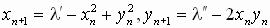Then, we introduce new variables x=x+by, h=x-by and parameters l1=l'+il", l2=l'-il", e=(1+b2)/4b2 and arrive at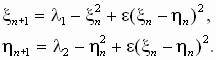These are two real coupled maps, with a rather special type of coupling. The next picture shows areas of different dynamics in the parameter plane (l1, l2) at several values of the coupling parameter e. At e>0.25 the region of finite motions corresponds precisely to a rotated and (in general) deformed Mandelbrot set.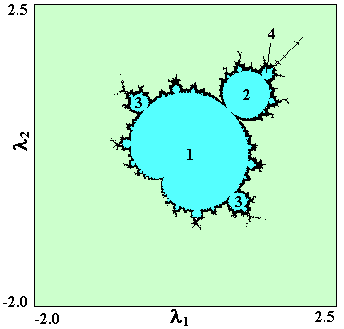e =0.5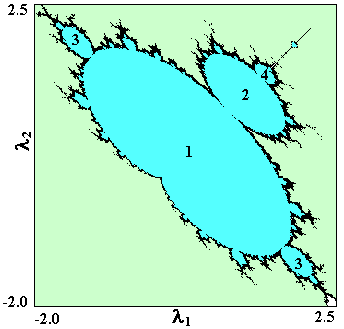e =0.3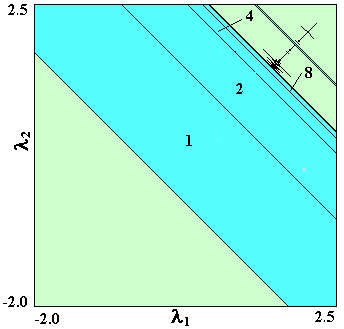e =0.25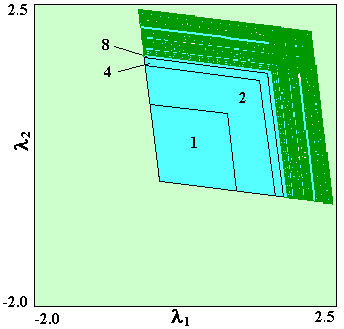e =0.1

The main receipt

 To build a physical system, in which the sets similar to those of Mandelbrot and Julia to be present, one can take two period-doubling systems and connect them in a manner properly chosen.
 How we can determine the required form of coupling? Let us take first an equation of an individual subsystem and set the dynamical variable complex. As well, the control parameter responsible for the period-doubling bifurcation cascade is regarded as complex. Next, we separate the real and imaginary parts in the equation and introduce symmetric and anti-symmetric variables. The result gives us equations of two coupled real systems of the required form.

Example #1. Coupled Hénon maps

In equationwe set z=x+iy, l=l'+il". Then,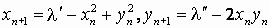Let us set x=x+by, h=x-by Ķ l1=l'+bl", l2=l'-bl", e=(1+b2)/4b2. As follows,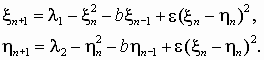The next figure shows regions of finite motions in such system at e=0.5 for several values of b. Although they differ visually from the Mandelbrot set, these are for sure "cactuses" of the same "race" (note presence of typical "bulbs", "antennas", etc.).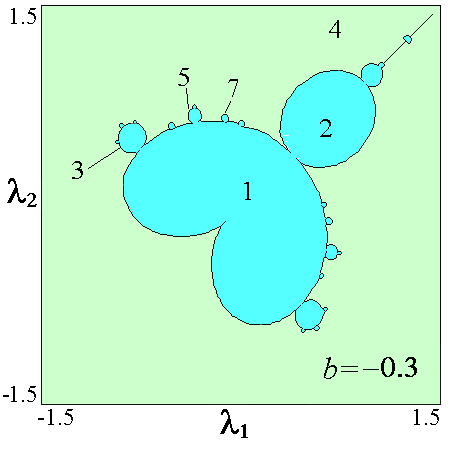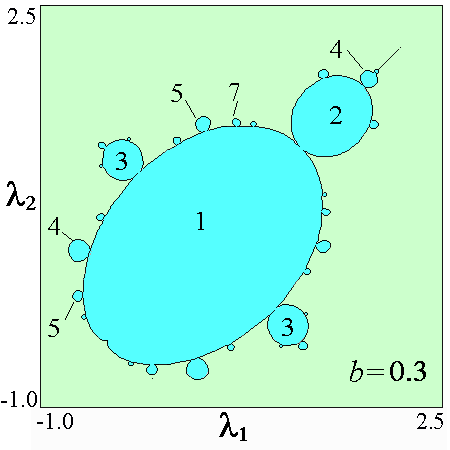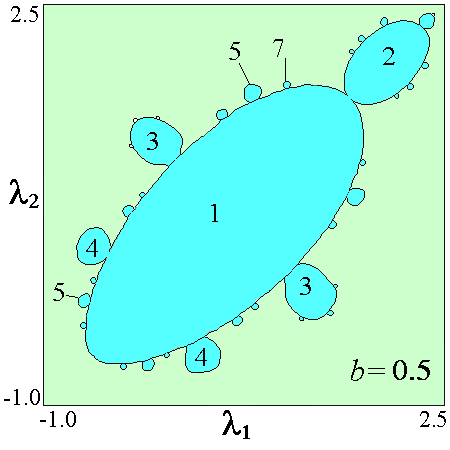Example #2. Coupled oscillators with quadratic nonlinearity driven by a periodic force

In equationlet us set z=x+iy, l=l'+il". Then,We set x=x+by, h=x-by and l1=l'+bl", l2=l'-bl", e=(1+b2)/4b2, to obtain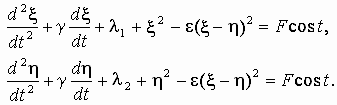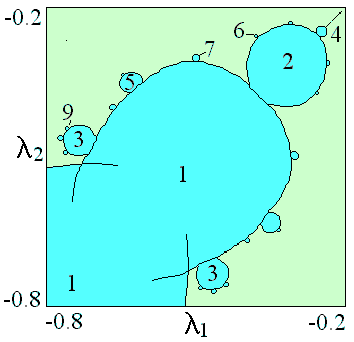Figure shows regions of finite motion in the parameter plane for the system of two coupled oscillators at e=0.5, g=0.2, F=0.23.

Observation of Mandelbrot set in a physical experiment

O.B.Isaeva, S.P.Kuznetsov, V.I.Ponomarenko. Mandelbrot Set in Coupled Logistic Maps and in Electronic Experiment. Phys.Rev E64, No 5, 2001, 055201.

A system representing a single real quadratic map consists of a pair of the sample-hold cells (in the shown schema they are marked by dotted frames and figures 1, 2). Each cell contains an switch and a capacitor. In the regime of picking a sample the capacitor is linked to the signal source via the switch, and accepts a charge up to a definite voltage. At some moment the switch breaks, and then the voltage on the capacitor remains constant - this is the regime of holding or storage. The voltage from the capacitor governs the operational amplifier of large input and low output resistance, so the charge of the capacitor remains practically constant. The used regime of the operational amplifier ensures equality of the output voltage to the input one. A set of two sample-hold cells is governed by two sequences of non-overlapped rectangular pulses: the switch K1 is opened while K2 is closed and vise versa. A multiplier N ensures quadratic nonlinearity to obtain squared value of the input voltage. A variable resistor R~ connected via the amplifier L gives a possibility to vary the control parameter l.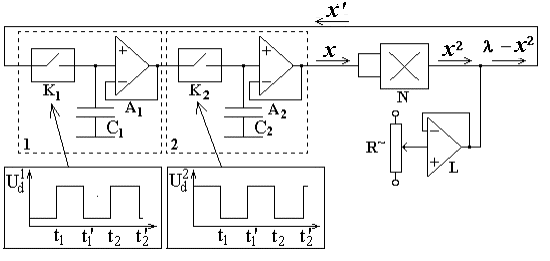In the experiment two such coupled subsystems were used, and coupling was arranged to produce a shift of control parameter l proportional to (x¢y)2.

Figures below show parameter plane charts obtained in the experiment at two values of the coupling parameter. In the first diagram one observes the Mandelbrot set (naturally, in the experiment it is possible to achieve a restricted resolution of the fine structure of the set). In the second diagram a romboid configuration is present that is typical for the situation of a small coupling.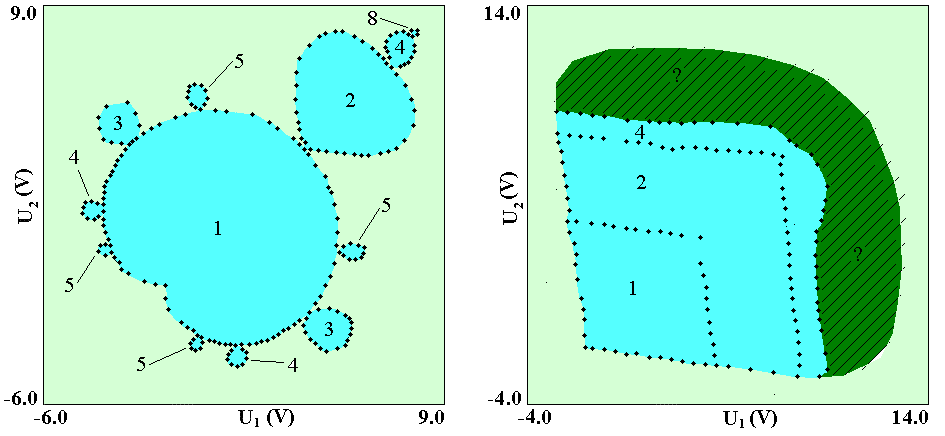ąźąŠčüčéąĖąĮą│ ąŠčé uCoz